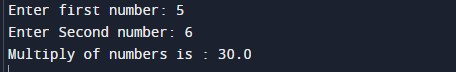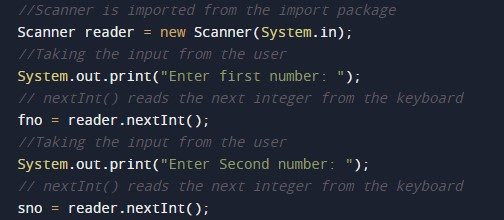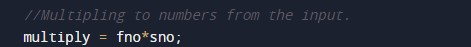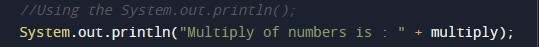# Java Program to Multiply two Floating Point Numbers

In this tutorial you will learn about the Java Program to Multiply two Floating Point Numbers and its application with practical example.

## Java Program to Multiply two Floating-Point Numbers

In this tutorial, we will learn to create a program that will multiply two Floating-Point Numbers in programming.

## Prerequisites:-

Before starting with this tutorial, we assume that you are the best aware of the following Java programming topics:

• Operators in Java Programming.
• Basic Input and Output function in Java Programming.
• Basic Java Programming.

## Program Description:-

As we all know, the integer, float, or double value in Java. First, we will take the input from the user in integer format. Secondly, we will multiply the numbers with the help of the arithmetic operator “*”. The numbers are the most useful terminology in any programming language.

With the help of this program, we can take input and Multiply Two Numbers.

## Output:-In the above program, we have first initialized the required variable.• fno = it will hold the input number float value from the user.
• sno = it will hold the input number float value from the user.
• multiply = it will hold the multiply value of the two numbers.

Taking input numbers from the user.Calculating the multiply of two numbers.Printing the output.In this tutorial we have learn about the Java Program to Multiply two Floating Point Numbers and its application with practical example. I hope you will like this tutorial.